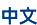Students would be enrolled under the first-level discipline Mathematics and receive the degree of MSc in Mathematics. They can choose from five pathways, or sub-disciplines: basic mathematics, computational mathematics, applied mathematics, probability theory and mathematical statistics, and operations research and control theory.

Overview of Doctoral Programs

Those who submit the required credentials and pass the examination would be enrolled. Successful doctoral candidates can choose from five pathways, sub-disciplines: basic mathematics, computational mathematics, probability theory and mathematical statistics, applied mathematics, and operations research and control theory.

Training types and schooling length

Fast-track master-doctor program: 5 years

Successive master-doctor program: 5 years in total

General doctoral program: 3 years

Introduction to the Five Pathways/Sub-disciplines

Basic Mathematics:

1. Lie group representation theory and automorphic forms, covering Lie group representation theory, automorphism, algebraic representation theory, number theory and their application.

2. Geometric analysis and topology, covering symplectic geometry, low-dimensional topology and algebraic geometry in mathematical physics, as well as important branches of differential geometry, including the study of Ricci curvatureminimal surfaceRicci flowmean curvature flow, etc.

3. Complex analysis and dynamical systems, covering the principle of minimal intersection of arithmetic dynamical systems, topological problems in complex dynamical systems, etc.

4. Real analysis and partial differential equations, covering Fourier analysis, harmonic analysis, well-posedness and stability theory of nonlinear partial differential equations, etc.

Computational Mathematics:

This discipline explores major issues in science and engineering. Students are guided to use computational methods to study the internal laws, and benefit the society through mathematical modeling, computational simulation, data processing, and process prediction.

The main research contents include mathematical theories, mathematical models, the design analysis and software realization of high-precision and high-efficiency structure-preserving algorithms.

Students are expected to become professionals in scientific and engineering calculations, inverse problems, artificial intelligence, machine learning, etc. with a solid theoretical foundation, deep mathematical understanding, a strong sense of innovation, and the ability of using mathematics and computer science to solve practical problems.

Probability Theory and Mathematical Statistics:

Probability theory and mathematical statistics has long been the advantageous discipline of Zhejiang University. It is led by Professor Lin Zhengyan and Professor Zhang Lixin, members of Institute of Mathematical Statistics. The research contents cover probability limit theory, random process orbit theory and statistical large sample theory, random matrix theory and method, non-stationary time series and high-dimensional spatial data theory and application, etc. Academic papers and teaching materials with international influence were published. Zhejiang University also boasts research in biostatistics and applied statistics, and achieved excellent results in the fields of dependent data models and adaptive randomized design of clinical trials.

Applied Mathematics:

This discipline cultivates professionals with a broad basic theory of mathematics. They would master the latest research results and development trends of mathematics and related subjects, and can independently engage in scientific research, apply mathematical theories to other subjects, and provide strategies for national development.

The main research directions include data science and artificial intelligence, medical image processing, application of partial differential equations, inverse problems in mathematical physics, algebraic coding, geometric design and computer graphics, fractal geometry, quantum computing, etc.

Operations Research and Control Theory:

This discipline cultivates professionals with the ability to use mathematics and computer theories based on systems and information processing. They should have independent scientific research capabilities to solve problems in system modeling analysis, planning, design, control and optimization in national strategic projects.

The main research directions include mathematical system theory, mathematical programming, distributed parameter system control theory, data analysis and decision optimization, combinatorial optimization, algorithm game theory, etc.